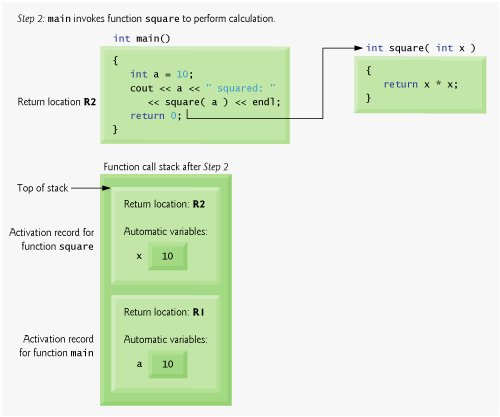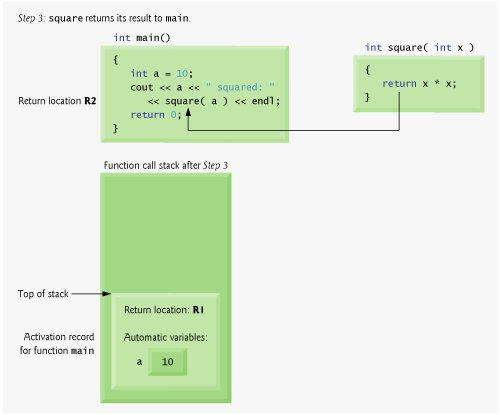# Function Call Stack and Activation Records

To understand how C++ performs function calls, we first need to consider a data structure (i.e., collection of related data items) known as a stack. Think of a stack as analogous to a pile of dishes. When a dish is placed on the pile, it is normally placed at the top (referred to as pushing the dish onto the stack). Similarly, when a dish is removed from the pile, it is normally removed from the top (referred to as popping the dish off the stack). Stacks are known as last-in, first-out (LIFO) data structuresthe last item pushed (inserted) on the stack is the first item popped (removed) from the stack.

One of the most important mechanisms for computer science students to understand is the function call stack (sometimes referred to as the program execution stack). This data structureworking "behind the scenes"supports the function call/return mechanism. It also supports the creation, maintenance and destruction of each called function's automatic variables. We explained the last-in, first-out (LIFO) behavior of stacks with our dish-stacking example. As we'll see in Figs. 6.146.16, this LIFO behavior is exactly what a function does when returning to the function that called it.

As each function is called, it may, in turn, call other functions, which may, in turn, call other functionsall before any of the functions returns. Each function eventually must return control to the function that called it. So, somehow, we must keep track of the return addresses that each function needs to return control to the function that called it. The function call stack is the perfect data structure for handling this information. Each time a function calls another function, an entry is pushed onto the stack. This entry, called a stack frame or an activation record, contains the return address that the called function needs to return to the calling function. It also contains some additional information we will soon discuss. If the called function returns, instead of calling another function before returning, the stack frame for the function call is popped, and control transfers to the return address in the popped stack frame.

The beauty of the call stack is that each called function always finds the information it needs to return to its caller at the top of the call stack. And, if a function makes a call to another function, a stack frame for the new function call is simply pushed onto the call stack. Thus, the return address required by the newly called function to return to its caller is now located at the top of the stack.

The stack frames have another important responsibility. Most functions have automatic variablesparameters and any local variables the function declares. Automatic variables need to exist while a function is executing. They need to remain active if the function makes calls to other functions. But when a called function returns to its caller, the called function's automatic variables need to "go away." The called function's stack frame is a perfect place to reserve the memory for the called function's automatic variables. That stack frame exists as long as the called function is active. When the called function returnsand no longer needs its local automatic variablesits stack frame is popped from the stack, and those local automatic variables are no longer known to the program.

Of course, the amount of memory in a computer is finite, so only a certain amount of memory can be used to store activation records on the function call stack. If more function calls occur than can have their activation records stored on the function call stack, an error known as stack overflow occurs.

Function Call Stack in Action

So, as we have seen, the call stack and activation records support the function call/return mechanism and the creation and destruction of automatic variables. Now let's consider how the call stack supports the operation of a square function called by main (lines 1117 of Fig. 6.13). First the operating system calls mainthis pushes an activation record onto the stack (shown in Fig. 6.14). The activation record tells main how to return to the operating system (i.e., transfer to return address R1) and contains the space for main's automatic variable (i.e., a, which is initialized to 10).

Figure 6.13. square function used to demonstrate the function call stack and activation records.

(This item is displayed on pages 269 - 270 in the print version)

``` 1 // Fig. 6.13: fig06_13.cpp
2 // square function used to demonstrate the function
3 // call stack and activation records.
4 #include
5 using std::cin;
6 using std::cout;
7 using std::endl;
8
9 int square( int ); // prototype for function square
10
11 int main()
12 {
13 int a = 10; // value to square (local automatic variable in main)
14
15 cout << a << " squared: " << square( a ) << endl; // display a squared
16 return 0; // indicate successful termination
17 } // end main
18
19 // returns the square of an integer
20 int square( int x ) // x is a local variable
21 {
22 return x * x; // calculate square and return result
23 } // end function square
```

 ``` 10 squared: 100 ```

Figure 6.14. Function call stack after the operating system invokes main to execute the application.

(This item is displayed on page 270 in the print version)Function mainbefore returning to the operating systemnow calls function square in line 15 of Fig. 6.13. This causes a stack frame for square (lines 2023) to be pushed onto the function call stack (Fig. 6.15). This stack frame contains the return address that square needs to return to main (i.e., R2) and the memory for square's automatic variable (i.e., x).

Figure 6.15. Function call stack after main invokes function square to perform the calculation.After square calculates the square of its argument, it needs to return to mainand no longer needs the memory for its automatic variable x. So the stack is poppedgiving square the return location in main (i.e., R2) and losing square's automatic variable. Figure 6.16 shows the function call stack after square's activation record has been popped.

Figure 6.16. Function call stack after function square returns to main.

(This item is displayed on page 272 in the print version)Function main now displays the result of calling square (line 15), then executes the return statement (line 16). This causes the activation record for main to be popped from the stack. This gives main the address it needs to return to the operating system (i.e., R1 in Fig. 6.14) and causes the memory for main's automatic variable (i.e., a) to become unavailable.

You have now seen how valuable the notion of the stack data structure is in implementing a key mechanism that supports program execution. Data structures have many important applications in computer science. We discuss stacks, queues, lists, trees and other data structures in Chapter 21, Data Structures, and Chapter 23, Standard Template Library (STL).C++ How to Program (5th Edition)
ISBN: 0131857576
EAN: 2147483647
Year: 2004
Pages: 627